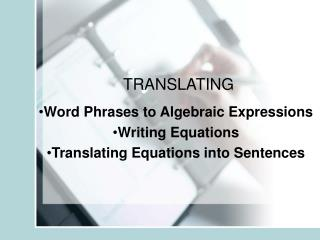DownloadDownload PresentationTRANSLATING

# TRANSLATING

Download Presentation## TRANSLATING

- - - - - - - - - - - - - - - - - - - - - - - - - - - E N D - - - - - - - - - - - - - - - - - - - - - - - - - - -
##### Presentation Transcript

1. TRANSLATING Word Phrases to Algebraic Expressions Writing Equations Translating Equations into Sentences

2. ADDITION Sum Total Added to Increased by More than SUBTRACTION Difference Subtracted from Decreased by Less than Phrases and symbols that suggest adding and subtracting.

3. MULTIPLICATION Product Times Multiplied by DIVISION Quotient Divided by Phrases and symbols that suggest multiplying and dividing. Try to stay away from using the symbol “X”. Why? Because it looks like a variable.

4. The quotient of (u + 2v) and w. The product of 1 and 13 Remember, stay away from the multiplication symbol “x” Write in symbols 1) Or 2)

5. 3) 19 less than w When using the Phrases More than less than Added to subtracted from TURN THE SYMBOLS AROUND 4) 13 subtracted from d 5) 10 meters added to the perimeter 6) Eight more than a number

6. Writing Equations and Formulas Words or phrases that suggest “ = “ Translating Equations Has 3 Steps Equals - Is - Is as much as - Is equal to - Is the same as - Is identical to 7) Nine times a number subtracted from 95 equals 37. Read the Words Define the Variable Let “n” represent “a number” Write the Equation

7. 3 Steps 8) Twice the number x decreased by eight equals seventy. Read the Words Let “x” represent “the number” Define the Variable Write the Equation Watch Out!! We’re not using the Less than or More than phrase So translate in order.

8. 9) The perimeter of a square equals four times the length of the side. F O R 10) The circumference (C) of a circle equals the product of 2, π, and the radius (r). M L A 11) The square of the hypotenuse (c) is equal to the sum of the squares of the legs (a) and (b). S

9. When translating from symbols into words, there is more than one way to translate an equation. 12) Translate into words the expression 4m – 19 • The product of 4 and a number “m” decreased by 19. • 4 times a number “m” minus 19. • 19 subtracted from 4 times a number “m” • The difference of 4 times a number “m” and 19.

10. 13) Translate into words the expression 3y + 15 = 14 14) Translate into words the expression 12 – 2x = -5 15) Translate into words the expression A = ½ bh